# Electromechanical Relay Logic

## Digital Circuits

• #### Question 1

In ladder logic symbolism, an electromechanical relay coil is shown as a circle, and the contact(s) actuated by the coil as two parallel lines, almost like a capacitor symbol. Given this knowledge, interpret the following ladder logic diagram: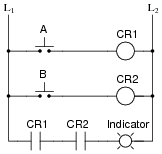How do we know which relay contact is actuated by which relay coil? How does this convention differ from that of standard electrical/electronic schematic diagrams, where the relay coil is shown as an actual coil of wire (inductor symbol) with the contact “linked” to the coil by a dashed line? Also, what type of logic function behavior (AND, OR, NAND, or NOR) does the above circuit exhibit?

• #### Question 2

There is a problem somewhere in this relay logic circuit. Lamp 2 operates exactly as it should, but lamp 1 never turns on. Identify all possible failures in the circuit that could cause this problem, and then explain how you would troubleshoot the problem as efficiently as possible (taking the least amount of electrical measurements to identify the specific problem).• #### Question 3

A very common application of electromechanical relay logic is motor control circuitry. Here is a ladder diagram for a simple DC motor control, where a momentary pushbutton switch starts the motor, and another pushbutton switch stops the motor:Translate this ladder diagram into point-to-point connections between the following components (shown in the following illustration):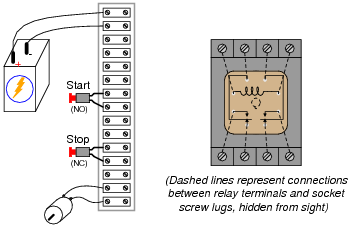• #### Question 4

Identify each of these relay logic functions by name (AND, OR, NOR, etc.) and complete their respective truth tables:• #### Question 5

Predict how the operation of this relay logic circuit will be affected as a result of the following faults. Consider each fault independently (i.e. one at a time, no multiple faults):Pushbutton switch A fails open:
Relay coil CR2 fails open:
Relay contact CR1-1 fails open:
Relay contact CR2-1 fails shorted:
Relay contact CR2-2 fails shorted:

For each of these conditions, explain why the resulting effects will occur.

• #### Question 6

Though many electronics students and professionals alike associate semiconductor components with the word “digital,” electromechanical relays are also digital logic (on or off) devices. In fact, some of the first digital computers were built with electromechanical relays as their active elements.

In what ways are electromechanical relays similar to semiconductor logic gates? In what ways do the two digital technologies differ?

• #### Question 7

The following schematic is of a relay circuit that emulates a standard digital logic gate function:Write a truth table for this circuit’s function, and determine what name best represents it (AND, OR, NAND, NOR, or NOT).

• #### Question 8

The following schematic is of a relay circuit that emulates a standard digital logic gate function:Write a truth table for this circuit’s function, and determine what name best represents it (AND, OR, NAND, NOR, or NOT).

• #### Question 9

A type of electrical diagram convention optimal for representing electromechanical relay circuits is the ladder logic diagram. An example of a “ladder logic” diagram is shown here:Each parallel circuit branch is represented as its own horizontal “rung” between the two vertical “rails” of the ladder. As you may have noticed, some of the symbols resemble standard electrical/electronic schematic symbols (toggle switches, for instance), while others are unique to ladder logic diagrams (heater elements, solenoid coils, lamps).

Where do the circuits shown obtain their electrical power? What do “L1” and “L2” represent? How are relay coils and contacts represented in a ladder logic diagram? Answer each of these questions by expanding upon the diagram shown above: draw the components necessary to show a complete electrical circuit (i.e. details of the power source), as well as an additional rung (or two) showing a relay coil actuated by some sort of switch contact, and the relay contact controlling power to a second indicator lamp.

• #### Question 10

Complete the following ladder logic diagram so that an OR gate function is formed: the indicator lamp energizes if either switch A or switch B is actuated.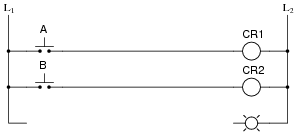• #### Question 11

In ladder logic diagrams, a normally-open relay contact is drawn as a set of parallel lines, almost like a non-polarized capacitor in an electronic schematic diagram. Normally-closed relay contacts differ in symbolism by having a diagonal line drawn through them.

Analyze the following relay logic circuit, completing the truth table accordingly:• #### Question 12

The following ladder logic diagram (for a steam heater control) contains a serious mistake:This is a mistake I’ve seen many students make. Explain what the mistake is, and draw a corrected version of this relay circuit.

• #### Question 13

Complete the truth table for the following relay logic circuit, and then complete a second truth table for the same circuit with relay coil CR2 failed open: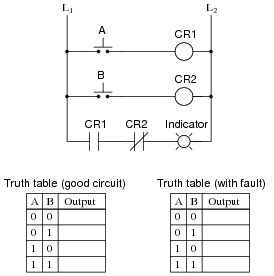Explain why the truth table will be modified as a result of the fault.

• #### Question 14

Predict how the operation of this motor control circuit will be affected as a result of the following faults. Consider each fault independently (i.e. one at a time, no multiple faults):“Stop” pushbutton switch fails open:
Relay contact CR1-1 fails open:
Relay contact CR1-2 fails open:
Relay coil CR1 fails open:

For each of these conditions, explain why the resulting effects will occur.

• #### Question 15

Suppose you come across a relay that is said to have “Form C” contacts. What does this phrase mean? And, is there such a thing as either “Form A” or “Form B” contacts?

• #### Question 16

Safety is a paramount concern in electrical systems. Generally, we try to design electrical circuits so that if and when they fail, they will do so in the manner safest to those people working around them, and to the equipment and process(es) controlled by the circuit.

One of the more common failure modes of circuits having wires strung through metal conduit is the accidental ground, or ground fault, where the electrical insulation surrounding a wire fails, resulting in contact between that wire and a grounded metal surface.

Suppose an accidental ground were to occur at the point shown in this ladder diagram: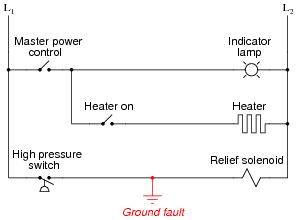What would be the result of this fault? Hint: you will need to know something about the L1/L2 power source in order to answer this question!

What would be the result if the L1/L2 power connections were reversed?

• #### Question 17

Complete the following ladder logic diagram so that an AND gate function is formed: the indicator lamp energizes if and only if both switch A and switch B are simultaneously actuated.• #### Question 18

Write a truth table for each of the indicator lamps in the following ladder diagram, and determine which logic function (AND, OR, NAND, NOR, or NOT) best describes each lamp’s behavior with respect to the status of the input switches.• #### Question 19

 Don’t just sit there! Build something!!

Learning to analyze relay circuits requires much study and practice. Typically, students practice by working through lots of sample problems and checking their answers against those provided by the textbook or the instructor. While this is good, there is a much better way.

You will learn much more by actually building and analyzing real circuits, letting your test equipment provide the “answers” instead of a book or another person. For successful circuit-building exercises, follow these steps:

1. Draw the schematic diagram for the relay circuit to be analyzed.
2. Carefully build this circuit on a breadboard or other convenient medium.
3. Check the accuracy of the circuit’s construction, following each wire to each connection point, and verifying these elements one-by-one on the diagram.
4. Analyze the circuit, determining all logic states for given input conditions.
5. Carefully measure those logic states, to verify the accuracy of your analysis.
6. If there are any errors, carefully check your circuit’s construction against the diagram, then carefully re-analyze the circuit and re-measure.

Always be sure that the power supply voltage levels are within specification for the relay coils you plan to use. I recommend using PC-board relays with coil voltages suitable for single-battery power (6 volt is good). Relay coils draw quite a bit more current than, say, semiconductor logic gates, so use a “lantern” size 6-volt battery for adequate operating life.

One way you can save time and reduce the possibility of error is, to begin with, a very simple circuit and incrementally add components to increase its complexity after each analysis, rather than building a whole new circuit for each practice problem. Another time-saving technique is to re-use the same components in a variety of different circuit configurations. This way, you won’t have to measure any component’s value more than once.